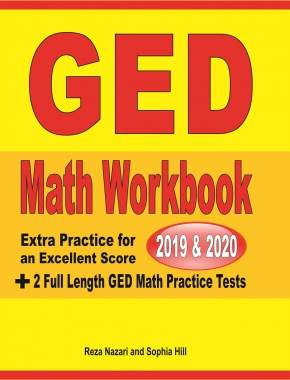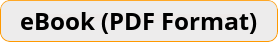\$15.99

# GED Math Workbook 2019 & 2020 Extra Practice for an Excellent Score + 2 Full Length GED Math Practice TestsLooking for a perfect GED Math workbook to help you prepare for the GED Math test? Need a great GED Math workbook for teaching, tutoring and preparing students for the GED Mathematical Reasoning test? If so, then this workbook is just for you.Teacher's Choice
100% Guaranteed
Secure Checkout

### A Perfect Workbook for the GED Mathematical Reasoning Test!

The best way to prepare for the GED Math Test is with intensive practice in every math concept tested–and that’s what you will get in GED Math Workbook 2019 & 2020.

This comprehensive workbook with more than 2,500 sample Math questions and two full length GED Mathematical Reasoning practice tests is all you need to measure your exam-readiness, find your weak areas, and learn from your mistakes.

Separate math chapters offer a comprehensive and complete review of the GED Math test, including:

• Arithmetic and Number Operations
• Algebra and Functions,
• Geometry and Measurement
• Data analysis, Statistics, & Probability
• … and also includes two full-length practice tests!

Written by GED Math experts and top GED Math instructors, this workbook left no stone unturned. The content of this book is 100% aligned with the 2019 and 2020 GED test guidelines.

ACE the GED Math Test with a PERFECT and COMPREHENSIVE Math Workbook!